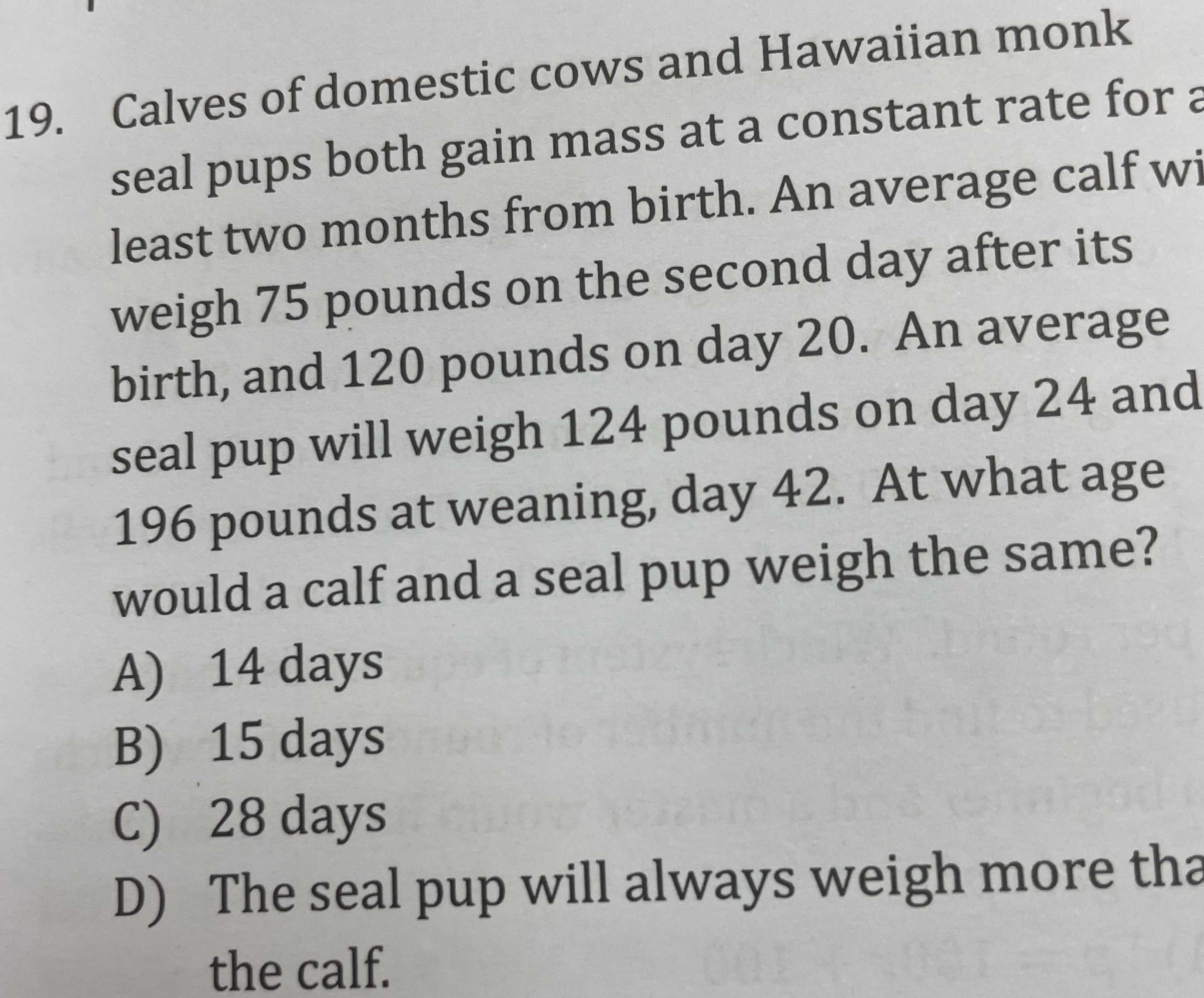### ¿Todavía tienes preguntas de matemáticas?

Pregunte a nuestros tutores expertos
Algebra
Pregunta19. Calves of domestic cows and Hawaiian monk seal pups both gain mass at a constant rate for a least two months from birth. An average calf wi weigh $$75$$ pounds on the second day after its birth, and $$120$$ pounds on day $$20$$ . An average seal pup will weigh $$124$$ pounds on day $$24$$ and

$$196$$ pounds at weaning, day $$42$$ . At what age would a calf and a seal pup weigh the same?

A) $$14$$ days

B) $$15$$ days

C) $$28$$ days

D) The seal pup will always weigh more tha the calf.